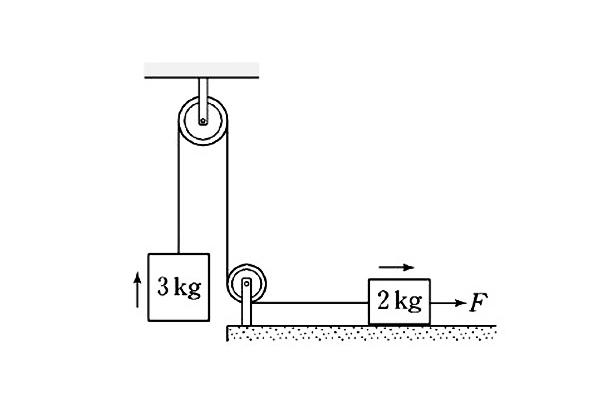# Kinetic friction forceWhen the force $F$ is acting toward the right on the 2 kg in the diagram, both objects are in uniform motion.

If the coefficient of kinetic friction between the 2 kg mass and the ground is $0.5,$ what is the magnitude of the force $F$ (in N)?

Gravitational acceleration is $g= 10 \text{ m/s}^2$.

×